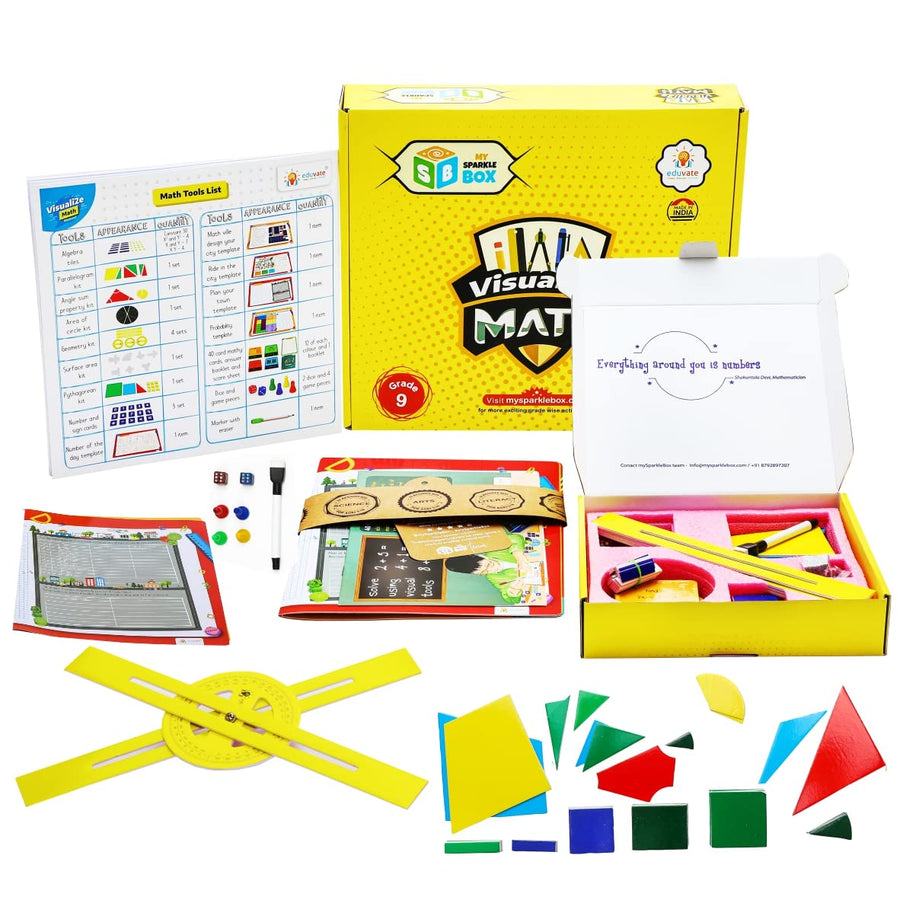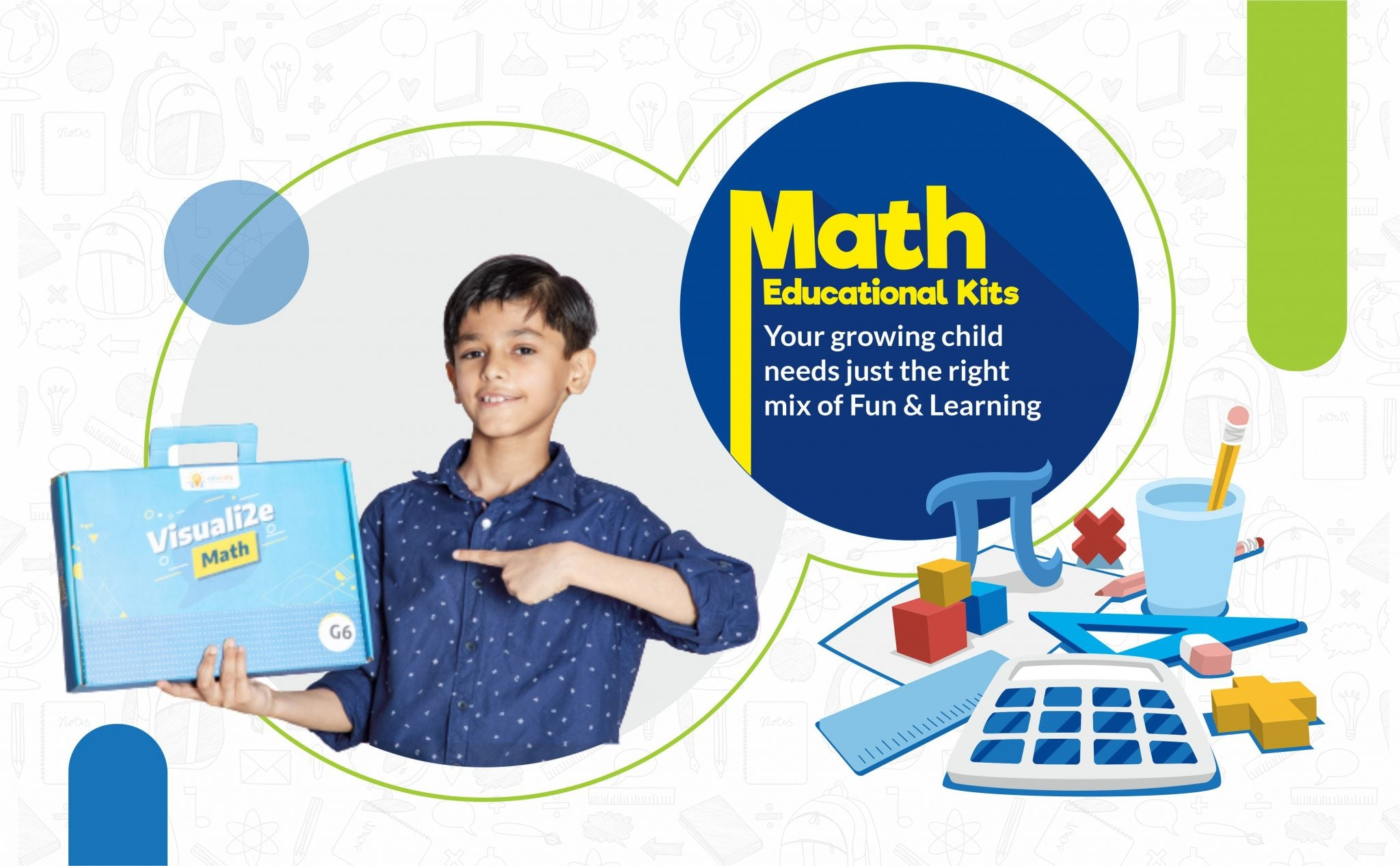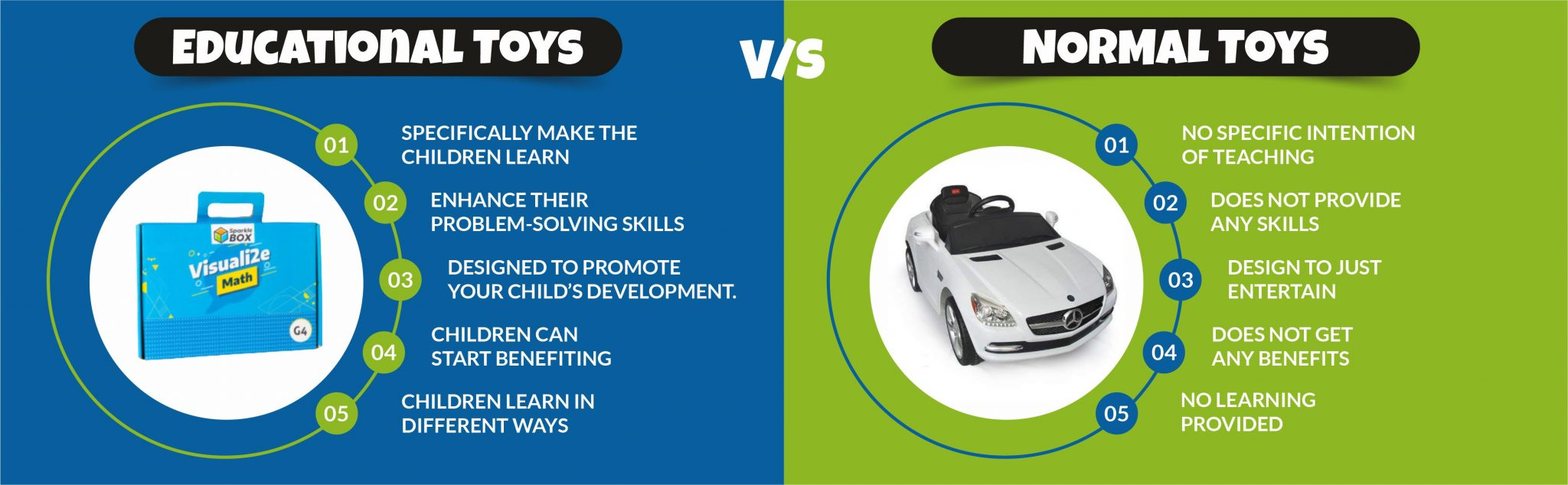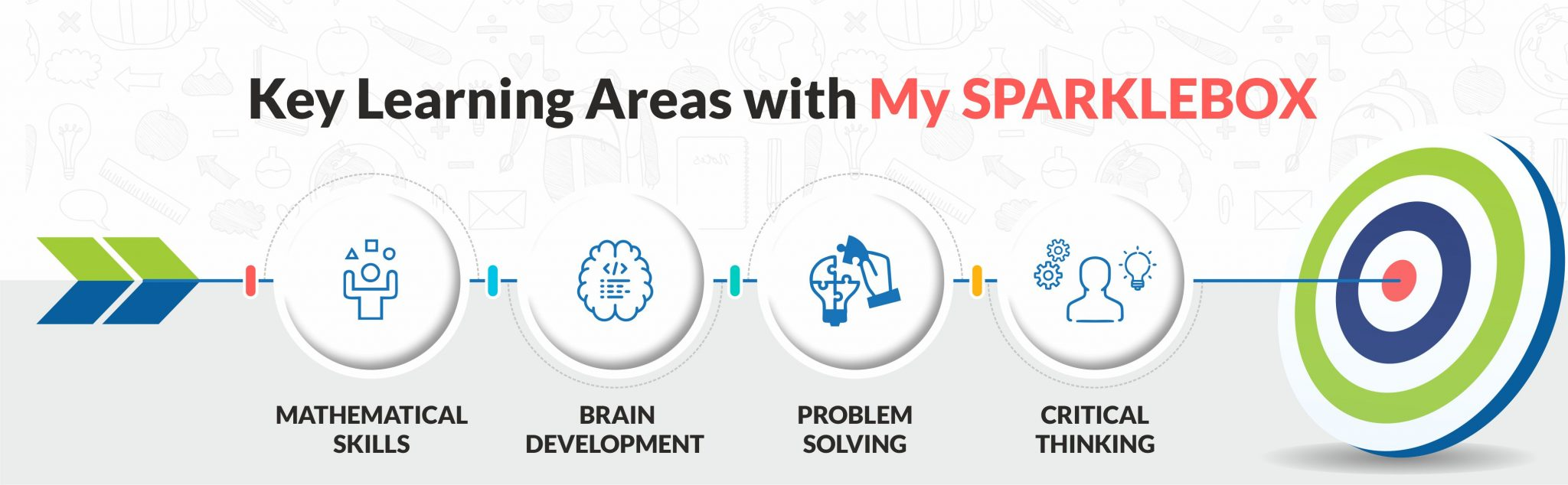DASARA SPECIAL OFFER!!! Get Extra 5% on and above the Existing Discount. Use Code: MSBDASARA05# SparkleBox Math Learning Kit for Grade 9 | 30+ Fun Activities | For kids aged 14 and above

Rating :

Regular price Rs. 1,326.00 Rs. 2,040.00

(35% Off)

Available:
In stock

SKU: JB21US012

Includes 30 fun activities | Make your Math learning stress free

What do you think of solving a linear equation, application of measurements, geometry, probability and algebra tiles? These are some of the concepts included in our activities and games that make the process of learning fun and stress-free.

Our activities and games are presented in such a way that it helps the child understand and learn the concepts while enjoying the process.

Math Learning Kit for Grade 9 provides an excellent learning opportunity to understand the concepts of the subject and provides a detailed manual with Concept wise outcomes and instructions for each activity.

INCLUDES FUN ACTIVITIES For HANDS-ON LEARNING | AGE: 12 years and above

SKILL SETS: Addition & Subtraction of like fractions, Multiplication & Division concepts, Arithmetic operations using currency, Word problem-solving techniques.INCLUDES FUN ACTIVITIES FOR HANDS ON LEARNING

Math Educational Toys & Kits to Make Mathematics Your Kid's FriendSparklebox Math Kit is an ideal gift, learning tool and an introductory kit for all basic mathematical concepts.

What should be in a math kit?

A maths kit for kids consists of number sequence, bonds, place value, odd and even numbers with reading, comprehending, and combination skills. Sparklebox’s math kit for children comes with all of this and much more.

How do you make math activities fun?

To make math activities fun for students, parents can involve their kids and explain to them the importance of math activities for kids and how all these applications will help them in the future with a core subject like maths. Sparklebox’s math kit comes with a wide range of play and learning activities to boost up the critical thinking of the children.

How do I make my kids smarter in math?

Old toys are no more fun. If you want your kids to become smarter in maths, introduce them to education kits and math activities for kids. Math activity is important for a kid in the growing years, as we all know how important math is. It is better to start early with Sparklebox’s math activity kits and toys.Concepts covered in the kit:

• Representation of algebra tiles
• Finding the value of the variable using algebra tiles
• Balancing algebraic equations using algebra tiles
• Addition of polynomials using algebra tiles
• Subtraction of polynomials using algebra tiles
• Multiplication of polynomials using algebra tiles
• Division of polynomials using algebra tiles
• Algebraic identities using algebra tiles
• Solving a linear equation in two variables using algebra tiles
• Factorization using algebra tiles
• Area of the circle using the area of circle kit
• Surface area and properties of solids using surface area kit
• Pythagoras theorem using a Pythagorean kit
• Area of a parallelogram using parallelogram kit
• Congruence of triangles using parallelogram kit
• Area of rhombus using parallelogram kit
• Area of trapezium using parallelogram kit
• Properties of a parallelogram using parallelogram kit
• Mid-point theorem using parallelogram kit
• Angle sum property and exterior angles using angle sum property kit
• Angles and types of angles using geometry kit
• Lines and angles using geometry kit
• Triangles and types of triangles using geometry kit
• Properties of quadrilaterals
• Playbook Template – Number of the day (application of operations on numbers)
• Playbook Template – Math ville: Design your city (application of measurements and geometry)
• Playbook Template – Ride in the city (application of ratio and proportion)
• Playbook Template – Plan your town (application of coordinate geometry)
• Playbook Template – Probability (application of probability)
• Game- 40 card mathy.

Components included in the kit:

• Constant 30, Square of X & Y -4, X & Y -7, XY-4
• 1 Set of Parallelogram Kit
• 1 Set Angel Sum Property Kit
• 1 Set of Area of Circle Kit
• 4 Set of Geometry Kit
• 1 Set of Surface Area Kit
• 1 Set of Pythagorean Kit
• 3 Set number and Sign cards
• 1 item, number of the day template
• 1 item, Math Ville, design your city template
• 1 item, ride in the city template
• 1 item, plan your town template
• 1 item probability template
• 10 of each color & 1 booklet, 40 Card Mathy answer booklet and score sheet
• 2 dice & 4 game pieces
• 1 item Marker

Includes 30 fun activities | Make your Math learning stress free

What do you think of solving a linear equation, application of measurements, geometry, probability and algebra tiles? These are some of the concepts included in our activities and games that make the process of learning fun and stress-free.

Our activities and games are presented in such a way that it helps the child understand and learn the concepts while enjoying the process.

## Customer Reviews

Based on 1 review
Related Products